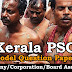## Recent Posts

1. Controlled oxidation of Methanol gives?
[a] Methanoic acid
[b] Methanol
[c] Carbon Dioxide and water
[d] Methane

2. New methods used in Chemistry which _____ to reduce pollution are called
[a] Organic Chemistry
[b] Polymer Chemistry
[c] Nano Technology
[d] Green Chemistry

3. The 7th State of matter _____?
[a] Quark Gluon Plasma
[b] Bose - Einsten Condensate
[c] Plasma
[d] Fermionic Condensate

4. The concept dual nature of matter was put forward by?
[a] I.H. Germer
[b] Louis de Broglie
[c] P.c. Roy
[d] Robert Boyle

5. The term atom was coined by?
[a] Ostwald
[b] Neils Bohr
[c] Dalton
[d] None of these

6. Law of multiple proportion was put forward by?
[a] Einstein
[b] Dalton
[c] Robert Boyle
[d] Lavoisier

7. The sub atomic particles of nucleus
[a] protons and electron
[b] electron only
[c] neutron and electron
[d] protons and neutrons

8. Lightest sub atomic particle is?
[a] Proton
[b] Neutron
[c] Electron
[d] Positron

9. The smallest atom is?
[a] Hydrogen
[b] Helium
[c] Francium
[d] Seasium

10. Who proposed the atomic theory?
[a] John Dalton
[b] Neils bohr
[d] Rutherford

11. Soda water contains
[a] Nitrous acid
[b] Sulphuric acid
[c] Carbon dioxide
[d] Hydrochloric acid

12. The name electron was proposed by?
[a] Louis - De - Broglie
[b] Rutherford
[c] Stoney
[d] Mossley

13. A solution of washing soda is?
[a] Acidic
[b] Basic
[c] Neutral
[d] None of these

14. The heaviest fundamental particle of an atom
[a] Electron
[b] Proton
[c] Neutron
[d] None of these

15. The atom without neutrons
[a] Deuterium
[b] Tritium
[c] Protium
[d] None of these

16. Structure of atom was first put forward by?
[a] Neils Bohr
[b] J.J. Thomson
[c] Dalton

17. The Chemical name of Baking Soda is?
[a] Sodium Hydroxide
[b] Sodium Carbonate
[c] Sodium Bicarbonate
[d] Sodium Nitrate

18. The maximum number of electrons in a shell?
[a] 2n
[b] 2n+1
[c] 2n-1
[d] 2n^2

19. The bricks of universe
[b] Molecules
[c] Atoms
[d] Electrons

20. The SI unit of the amount of substance
[a] Mole
[b] Ampere
[c] Candela
[d] Kelvin

Model Question Paper Company Corporation Board Assistant - 54Reviewed by Santhosh Nair on 2:04 PM Rating: 5